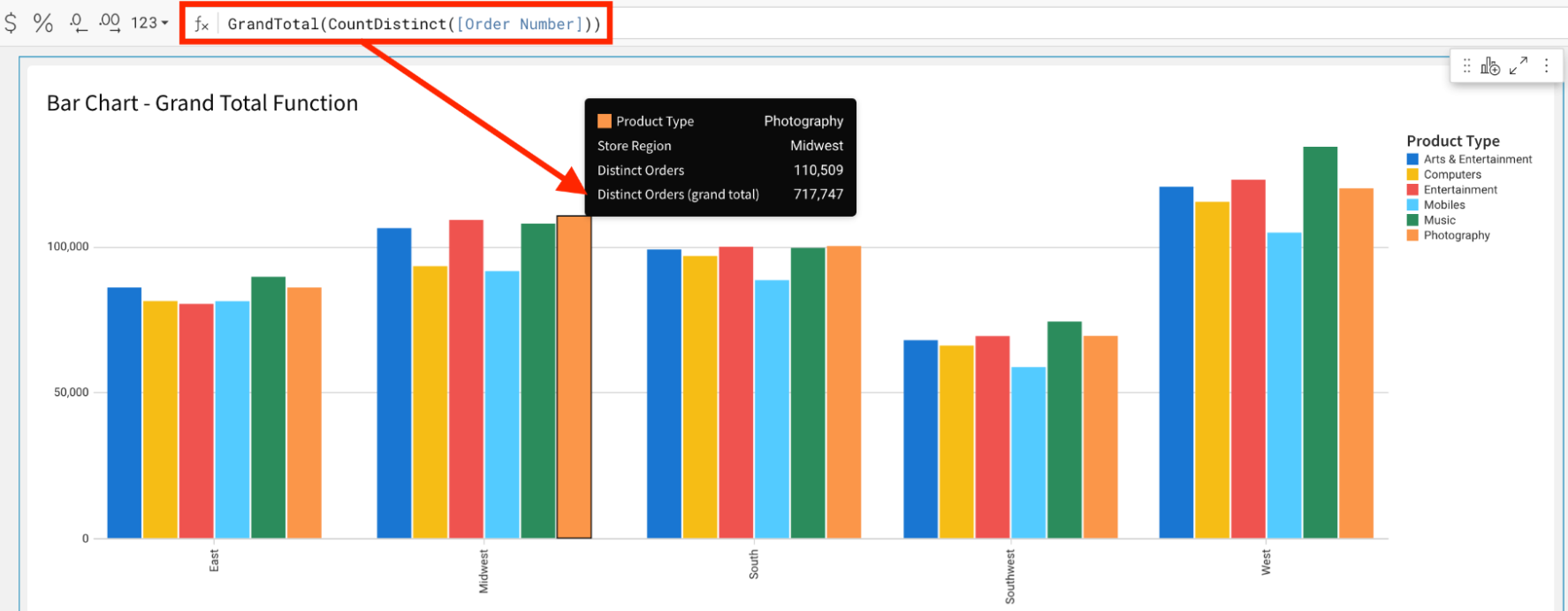# GrandTotal

The GrandTotal formula returns the grand total for the given aggregate formula. GrandTotal is shorthand for Subtotal(aggregate, "grand_total").

## Syntax

`GrandTotal(aggregate)`

Function arguments:

• aggregate (required) - The aggregate formula to use in the function.
• Examples: `Count(), Sum([Sales Amount])`

## Example

In a chart visualizing order counts per Product Type and Region, you can add a calculated column with the formula to the tooltip:

`GrandTotal(CountDistinct([Product Type]))`

To see an overall total count of distinct orders across all categories.

• The calculation is shown in the tooltip in this example, but is usable anywhere an aggregate formula is allowed.